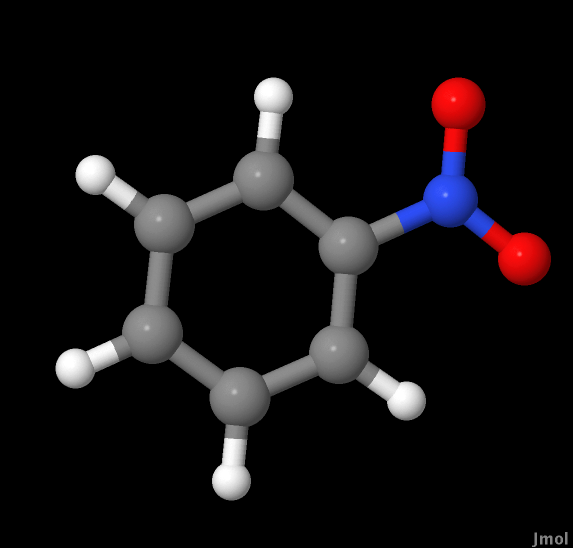Molecular Orbital Calculations on CO, CO2 and Nitrobenzene
by Alex Dombeck, Kim Wallace and Luke Whitman

Introduction

To obtain an understanding of the reactivity of a molecule, the electronic structure of a species can be examined and used to locate the probable location of electron density. Once this information is determined, other properties of a molecule can be predicted and used to understand reactivity. Properties that can be calculated include dipole moment and polarizability, frequency of vibration, and electron donating character.

The calculations required to obtain this information about electronic structure are complicated and require a high-level understanding of quantum mechanics. To assist in these calculations and reduce time intensiveness, software programs that utilize several approximation methods are being developed by scientists. These methods rely on a principle called the variation method. In summary, the variation principle says that the approximations with the lowest energy are the ones closest to the real physical quantities.

Using these approximation methods allows for calculations to be performed for complicated molecules that are not solvable analytically. Classical mechanics can be used with the program Molecular Mechanics, which yields low-accuracy values. Higher accuracy approximations require the use of software with capabilities for performing molecular mechanic calculations. Software programs such as GAMESS, can be used to assist in these calculations. Two methods of approximation, semi-emperical and ab initio, will be completed using GAMESS. Using some empirical data, programs such as MOPAC can be used to calculate semi-empirical values of overlap integrals. Ab initio theory calculations are the best approximations for molecular mechanics. They utilize Hartree Fock Self-Consistent Field model and a manually selected basis set size. Although there is some inherent approximation, there are various basis sets available to improve the accuracy of the approximations.

After performing calculations for the electronic structure of a molecule and obtaining approximations for the highest level of theory, molecular properties will be calculated and compared to empirical values.

Experimental

Calculations were performed using software available on the UW-Oshkosh quantum server in order to obtain molecular mechanics approximations for the following molecules: Carbon Monoxide, Carbon Dioxide, and Nitrobenzene. Initial geometries for molecules were configured using wxMacMolPlt and were saved as “.cml” files. These files were opened using Jmol and a molecular mechanic optimization was performed for each molecule. Files were then saved as “.xyz” files. The molecular mechanic optimized structures were used to generate AM1 and PM3 geometry optimized “.inp” files, which were submitted to GAMESS using GamessQ. Molecules with completed calculations were verified as being correctly calculated by evaluating the logs for each and saving geometries for completed calculations with “.log” extension. These geometries were viewed using wxMacMolPlt. After all calculations for geometries were completed for these levels of theory, the dipole moment for each molecule and level of theory was obtained from “.log” files.

The potential energy surface versus bond length was calculated for each molecule using the best ab initio result and the following basis sets: 6-21G, 6-31G and DZV. The resulting approximations were confirmed as being completed by examining the log files and were saved. From the log file for each molecule and level of theory, the vibrational frequencies for each were extracted and compared to literature values.

A final calculation was performed for only Nitrobenzene. From the log file for each level of theory, the expected peaks for a UV-Vis spectra were calculated. These values were compared with an experimental spectrum for Nitrobenzene.

 Carbon Monoxide Carbon Dioxide NitrobenzeneVibrational information on carbon dioxideVibrational information on nitrobenzene

Conclusion
This laboratory demonstrated how computational results could be used to determine the molecular geometry of molecules and make hypothesis for other properties of molecules, including dipoles, vibrational frequencies, and spectral absorption peaks. A scientist with ample understanding of the calculations being computed must perform these calculations and the results of these calculations must be analyzed carefully. For example, this software is extremely useful for predicting the molecular geometries and preparing diagrams for molecular orbitals; however, the data obtained for some properties of the molecules was less accurate for some levels of theory. An example of discrepancies between calculated values and theoretical values could be seen in the calculations of dipole moments. High level theory calculations were not close to literature values for dipole for carbon monoxide. Another example is the UV-Vis spectrum for nitrobenzene. Peaks were calculated for this molecule but did not appear in literature spectra.

Computational calculations can be useful for some purposes and can save time when compared to doing the calculations by hand; however, the results of these calculations must be analyzed with caution. These computations can be useful for understating the orbitals of molecules but are not useful for calculating values for dipole moments and obtaining spectral data. Literature references can be used to cross check the values obtained by these calculations to ensure that calculations are useful.

References:
(1)  Mihalick, J.; Gutow, J. Molecular Orbital (MO) Calculations. Oshkosh, WI, 2011.

(2) National Institute of Standards and Technology. NIST Chemistry WebBook; http://webbook.nist.gov/chemistry/, (accessed 3/7/11).